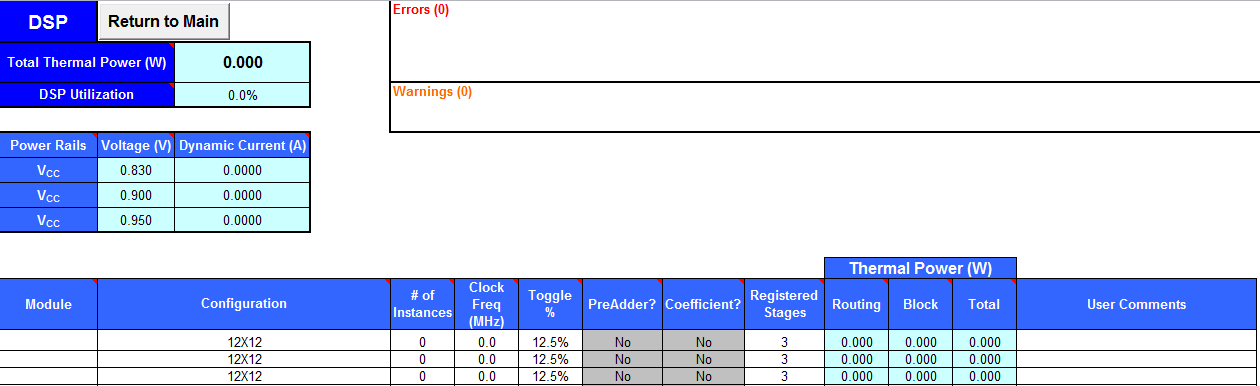ID 683688
Date 9/27/2022
Public
Give Feedback

## 4.5. Arria® 10 EPE - DSP Worksheet

Each row in the DSP worksheet of the Early Power Estimator (EPE) for Arria® 10 represents a DSP design module where all instances have the same configuration, clock frequency, toggle percentage, and register usage.
Figure 15. DSP Worksheet of the Early Power EstimatorTable 10.  DSP Worksheet Information
Module

Enter a name for the DSP module in this column. This is an optional value.

Configuration Select the DSP block configuration for the module.
# of Instances

Enter the number of DSP block instances that have the same configuration, clock frequency, toggle percentage, and register usage. This value is not necessarily equal to the number of dedicated DSP blocks you use. For example, it is possible to use two 18 × 18 simple multipliers that are implemented in the same DSP block in the FPGA devices. In this case, the number of instances would be two.

To determine the maximum number of instances you can fit in the device for any particular mode, follow these steps:

1. Open the Variable Precision DSP Blocks chapter of the Arria® 10 Device Handbook.
2. In the Number of DSP Blocks table, take the maximum number of DSP blocks available in the device for the mode of operation.
3. Divide the maximum number by the # of Mults for that mode of operation from the DSP Block Operation Modes table. The resulting value is the maximum number of instances supported by the device.
Clock Freq (MHz)

Enter the clock frequency for the module (in MHz). This value is limited by the maximum frequency specification for the device family.

Toggle %

Enter the average percentage of DSP data outputs toggling on each clock cycle. The toggle percentage ranges from 0 to 50%. The default value is 12.5%. For a more conservative power estimate, use a higher toggle percentage.

50% corresponds to a randomly changing signal, since half the time the signal will hold the same value and thus not transition. This is considered the highest meaningful toggle rate for a DSP block.

Preadder? Select Yes if the PreAdder function of the DSP block is turned on.
Coefficient? Select Yes if the Coefficient function of the DSP block is turned on.
Registered Stages

Select number of the registered stages. Having more stages registered increases DSP fMAX and reduces power consumption at the cost of increased latency.

• 0—None
• 1—Input
• 2—Input and Output
• 3—Input, Output, and Multiplier
• 4—Input, Output, Multiplier, and Floating-Point Adder
Thermal Power (W)–Routing

Indicates the power dissipation due to estimated routing (in W).

Routing power depends on placement and routing, which is a function of design complexity. The values shown represent the routing power estimate based on observed behavior across more than 100 real-world designs.

Thermal Power (W)–Block

Indicates the estimated power consumed by the DSP blocks (in W).

Thermal Power (W)–Total

Indicates the estimated power (in W), based on information entered into the EPE spreadsheet. It is the total power consumed by the DSP blocks and is equal to the routing power and block power.